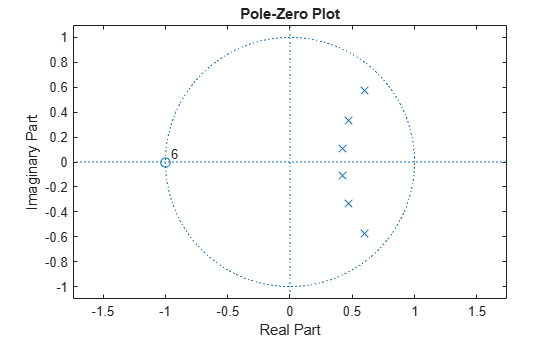# isminphase

Determine whether filter is minimum phase

## Syntax

```flag = isminphase(b,a) flag = isminphase(sos) flag = isminphase(d) flag = isminphase(...,tol) ```

## Description

`flag = isminphase(b,a)` returns a logical output, `flag`, equal to `true` if the filter specified by numerator coefficients, `b`, and denominator coefficients, `a`, is a minimum phase filter.

`flag = isminphase(sos)` returns `true` if the filter specified by second order sections matrix, `sos`, is minimum phase. `sos` is a K-by-6 matrix, where the number of sections, K, must be greater than or equal to 2. Each row of `sos` corresponds to the coefficients of a second order (biquad) filter. The ith row of the `sos` matrix corresponds to ```[bi(1) bi(2) bi(3) ai(1) ai(2) ai(3)]```.

`flag = isminphase(d)` returns `true` if the digital filter, `d`, has minimum phase. Use `designfilt` to generate `d` based on frequency-response specifications.

`flag = isminphase(...,tol)` uses the tolerance, `tol`, to determine when two numbers are close enough to be considered equal. If not specified, `tol`, defaults to `eps^(2/3)`.

## Examples

collapse all

Design a sixth-order lowpass Butterworth IIR filter using second order sections. Specify a normalized 3-dB frequency of 0.15. Check if the filter has minimum phase.

```[z,p,k] = butter(6,0.15); SOS = zp2sos(z,p,k); min_flag = isminphase(SOS)```
```min_flag = logical 1 ```

Redesign the filter using `designfilt`. Check that the zeros and poles of the transfer function are on or within the unit circle.

```d = designfilt('lowpassiir','DesignMethod','butter','FilterOrder',6, ... 'HalfPowerFrequency',0.25); d_flag = isminphase(d)```
```d_flag = logical 1 ```
`zplane(d)`Given a filter defined with a set of single-precision numerator and denominator coefficients, check if it has minimum phase for different tolerance values.

```b = single([1 1.00001]); a = single([1 0.45]); min_flag1 = isminphase(b,a) ```
```min_flag1 = logical 0 ```
`min_flag2 = isminphase(b,a,1e-3)`
```min_flag2 = logical 1 ```